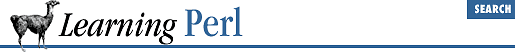home | O'Reilly's CD bookshelfs | FreeBSD | Linux | Cisco | Cisco Exam

### 4.6 Exercises

1. Write a program that asks for the temperature outside, and prints "too hot" if the temperature is above 72, and "too cold" otherwise.

2. Modify the program from the previous exercise so that it prints "too hot" if the temperature is above 75, "too cold" if the temperature is below 68, and "just right!" if it is between 68 and 75.

3. Write a program that reads a list of numbers (on separate lines) until the number ``` 999``` is read, and then prints the total of all the numbers added together. (Be sure not to add in the ``` 999``` !) For example, if you enter ``` 1``` , ``` 2``` , ``` 3``` , and ``` 999``` , the program should reply with the answer of ``` 6``` (``` 1+2+3``` ).

4. Write a program that reads in a list of strings on separate lines and then prints out the list of strings in reverse order - without using ``` reverse``` on the list. (Recall that ``` <STDIN>``` will read a list of strings on separate lines when used in an array context.)

5. Write a program that prints a table of numbers and their squares from zero to 32. Try to come up with a way where you don't need to have all the numbers from 0 to 32 in a list, and then try one where you do. (For nice looking output,

```

```
printf "%5g %8g\n", \$a, \$b```
```

prints ``` \$a``` as a five-column number and ``` \$b``` as an eight-column number.)4.5 The foreach Statement5. Hashes